Question

Simplify the following expression.

\displaystyle{\frac{4x+14}{2}\div\frac{4x+14}{x-6}}

1.thnahdat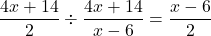General Formulas and Concepts:

Pre-Algebra

Order of Operations: BPEMDAS

1. Brackets
2. Parenthesis
3. Exponents
4. Multiplication
5. Division
7. Subtraction
• Left to Right

Dividing Fractions – KCF (Keep Change Flip)

• Keep the 1st fraction the same
• Change the sign from division to multiplication
• Flip the 2nd fraction (reciprocate)

Algebra I

• Terms/Coefficients
• Domains

Step-by-step explanation:

Step 1: Define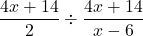Step 2: Simplify

1. Divide [KCF]: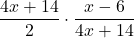2. Multiply: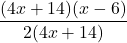3. Divide: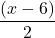Extra:

If we were to graph this, we would need to watch out for domain restrictions or changes because we are combining 2 domains together when 1 of them has a restriction.

2.